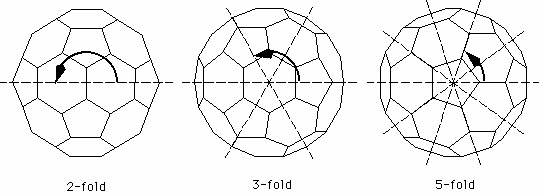Max-Planck-Institut für Festkörperforschung Andersen Group LMTO C60 GW Clusters

## Symmetry of the C60 molecule

The C60 molecule is highly symmetric, that means one can find many transformations that map the molecule onto itself. All such symmetry operations for a molecule are rotations around an axis, reflections in a plane, and sometimes so called inversions. Clearly all symmetry operations must leave the center of mass of the molecule in place, so all rotation axes and mirror planes must go through that point.

For the C60 molecule there are three kinds of rotation axes. The most obvious ones are the 5-fold axes through the centers of two facing pentagons. Look down on one of the pentagons and you see that the molecule is symmetric under rotations of 360/5 = 72 degrees. Next there are a rotation axes through the center of two facing hexagons. Observe that these axes are only 3-fold, i.e. it takes a rotation of 120 degrees to map the molecule onto itself. Finally there are 2-fold axis through the centers of the edges between two hexagons.Rotation axes of the C60 molecule

Since there are 12 pentagons, there are 6 different 5-fold axes (remember that each axis passes through two pentagons). Likewise, since there are 20 hexagons, there are 10 different threefold axes. To find the number of different two-fold axes, observe that each hexagon is neighboured by three other hexagons. Hence there are 30 edges between two hexagons, i.e. 15 different 2-fold axes.

The reflections symmetries are also related to the edges between adjacent hexagons: The mirror planes contain two such edges, hence there are also 15 different mirror planes.

Finally, the C60 molecule is invariant under the inversion with respect to the center of mass. This means that if you replace each point with coordinates (x,y,z) by (-x,-y,-z), the molecule is mapped onto itself.

Combining all those transformations, one finds 120 different symmetry operations. They form the icosahedral group which is the point group with the largest number of elements. Hence C60 can be called the most symmetric molecule.

 MPI-FKF Andersen Group Max-Planck-Institut für Festkörperforschung Heisenbergstraße 1 D-70569 Stuttgart